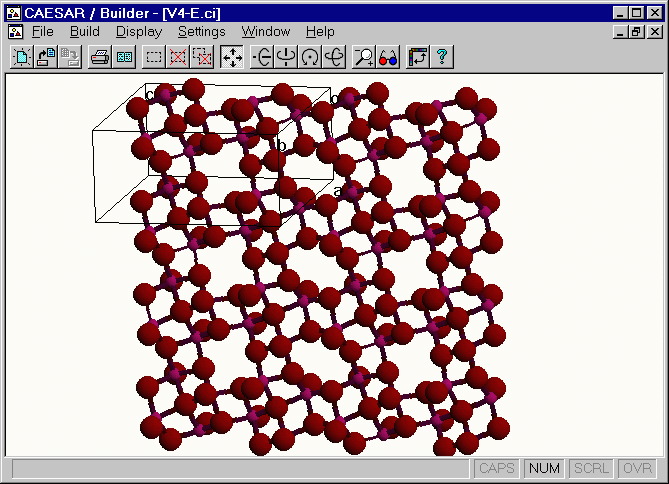# Crystal Structure of V4O9(-2) Layer

The [H2N(CH2)4NH2]V4O9 salt has two equivalent [V4O9]2- layers per unit cell.13 A perspective view of one [V4O9]2- layer, which was obtained by using the v4-o.CI file in the CAESAR\Examples\Chapter5\AV4O9 directory. This layer has four different vanadium atoms V(1), V(2), V(3) and V(4), each V atom is in a square-pyramidal coordinate, and the bond valence sum analysis 14 for each VO5 unit shows that the oxidation state of each V atom is V4+ (d1).

The magnetic susceptibility-versus-temperature (c-vs.-T) curve for the [H2N(CH2)4NH2] V4O9 salt shows that the spins of the V4+ ions interact antiferromagnetically. The c-vs.- T plot has a maximum at 30 K (i.e., cmax = 0.011 emu/mol at Tmax = 30 K), but the decrease of c in the temperature region above Tmax is not sharp.13 To understand this general feature of the c-vs.- T plot, it is necessary to examine how the nearest-neighbor spins interact in the [V4O9]2- layer.Figure. Extended view of the [V4O9]2- layer.

Go back to The Gallery